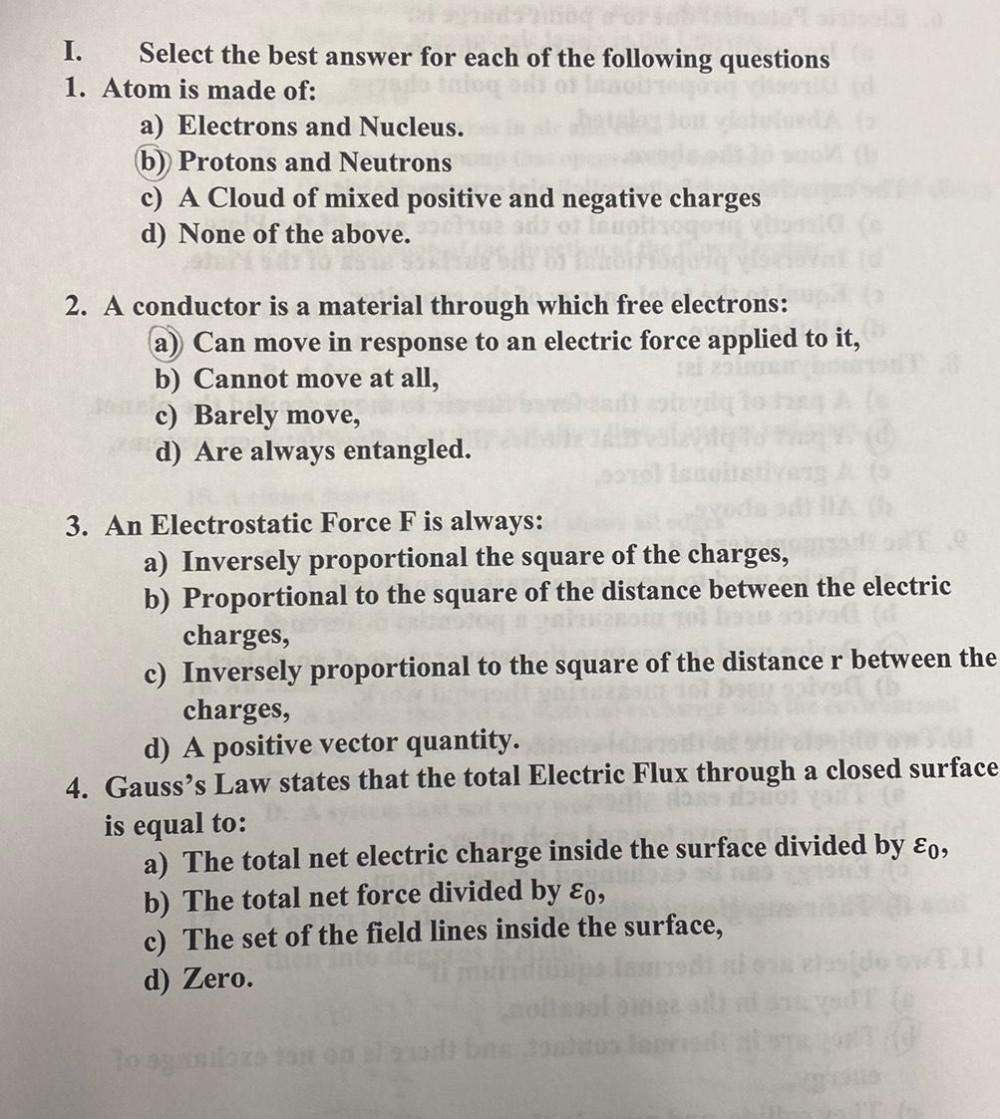Question:

# I. Select the best answer for each of the following questions 1. Atom is made of: a) Electrons and Nucleus. b) Protons and NeutrI. Select the best answer for each of the following questions 1. Atom is made of: a) Electrons and Nucleus. b) Protons and Neutrons c) A Cloud of mixed positive and negative charges d) None of the above. 2. A conductor is a material through which free electrons: a) Can move in response to an electric force applied to it, b) Cannot move at all, c) Barely move, d) Are always entangled. 3. An Electrostatic Force F is always: a) Inversely proportional the square of the charges, b) Proportional to the square of the distance between the electric charges, c) Inversely proportional to the square of the distance r between the charges, d) A positive vector quantity. 4. Gauss's Law states that the total Electric Flux through a closed surface is equal to: a) The total net electric charge inside the surface divided by £o, b) The total net force divided by Eo, c) The set of the field lines inside the surface, d) Zero. To